The Pythagorean Theorem: The Way of Truth

Articleby
published on 22 February 2011

Pythagoras (569-475 BC) is recognized as the world’s first mathematician. He was born on the island of Samos and was thought to study with Thales and Anaximander (recognized as the first western philosophers). Pythagoras believed that numbers were not only the way to truth, but truth itself. Through mathematics, one could attain harmony and live an easier life. He is said to have proposed a number of mathematical theorems to this end but, of all these, only the famous Pythagorean Theorem remains (Allen, 1966).

The historian Robinson writes, “The statement that `Pythagoras worked very hard at the arithmetical side of geometry’ is further borne out by the tradition that he investigated the arithmetical problem of finding triangles having the square on one side equal to the sum of the squares on the other two” and did so, early on, by using stones in rows to understand the truths he was trying to convey (1968). The Pythagorean Theorem states that a² + b² = c². This is used when we are given a triangle in which we only know the length of two of the three sides. C is the longest side of the angle known as the hypotenuse. If a is the adjacent angle then b is the opposite side. If b is the adjacent angle then a is the opposite side. If a = 3, and b = 4, we could then solve for c. 32 + 42 = c². 9 + 16 = c². 25 = c². c = 5. This is one of the prime uses of the Pythagorean Theorem.

There are many proofs of the Pythagorean Theorem, the best known being Euclid’s proof from Book I of his Elements.

Proposition: In right-angled triangles the square on the hypotenuse is equal to the sum of the squares on the legs.

Euclid started with a Pythagorean configuration and then drew a line through a diagram illustrating the equalities of the areas. He concluded that AB/AC = AC/HA, therefore (AC)² = (HA)(AB). Since AB=AJ, the area of the rectangle HAJG corresponds to the area of the square on side AC. Similarly, AB/BC = BC/BH also written as (BC)² = (BH)(AB) = (BH)(BD) and since AB=BD. Thus we see that the sum of the areas of the rectangles is the area of the square on the hypotenuse. In the words of Stephanie Morris, “This completes the proof” (Morris, 2011).

Another proof, which is easier for people to understand, starts off with a rectangle divided into three triangles, all with right angles.

Triangle BEA and triangle BCE overlap triangle ACD. Comparing triangle BCE and triangle ACD, and looking at their corresponding sides, we see that AC/BC = AD/EC. Since AD = BC, AC/AD = AD/EC. Through multiplication this equation is rendered (AD)² = (AC)(AE). From triangles ABC and ABE, noting that AB = CD, comparing the right angles of these two figures we render the equation AC/AB = CD/AE. From the original rectangle shape we had AB = CD also given as AC/CD = CD/AE, which is written as a multiplication problem as (CD)² = (AC)(AE) and by adding the equations we have so far, we obtain two new formulas which are (CD)² + (AD)² = (AC)(AE) + (AC) (EC) and (CD)² + (AD)² = (AC)(AE + EC). Since AC = AE + EC, we obtain (CD)² + (AD)² = (AC)². As with the earlier proof, this shows the validity of the Pythagorean Theorem (Morris, 2011).

In the Pythagorean Theorem every side/angle is a critical piece of information that helps us determine other angles/sides. Pythagoras believed in an objective truth which was number. The Pythagorean Theorem allows for truths to be known through the mathematical equations above which means that there does exist an objective truth, outside of any personal opinion, which can actually be proven; and this, finally, is what Pythagoras wanted to prove through his work.

Editorial Review This Article has been reviewed for accuracy, reliability and adherence to academic standards prior to publication.

Recommended Books

•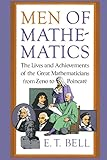E.T. Bell Touchstone (15 October 1986) Price: \$18.00
•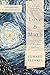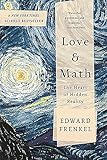Edward Frenkel Basic Books (09 September 2014) Price: \$15.28
•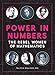Talithia Williams Race Point Publishing (08 May 2018) Price: \$6.52
•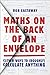Rob Eastaway HarperCollins (17 October 2019) Price: \$13.54
•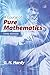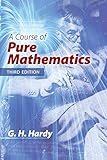G. H. Hardy Dover Publications (18 July 2018) Price: \$22.80

Cite This Work

APA Style

Bernholc, E. D. (2011, February 22). The Pythagorean Theorem: The Way of Truth. Ancient History Encyclopedia. Retrieved from https://www.ancient.eu/article/213/

Chicago Style

Bernholc, Eric D. "The Pythagorean Theorem: The Way of Truth." Ancient History Encyclopedia. Last modified February 22, 2011. https://www.ancient.eu/article/213/.

MLA Style

Bernholc, Eric D. "The Pythagorean Theorem: The Way of Truth." Ancient History Encyclopedia. Ancient History Encyclopedia, 22 Feb 2011. Web. 16 Oct 2019.Our latest articles delivered to your inbox, once a week:

Are you a...?